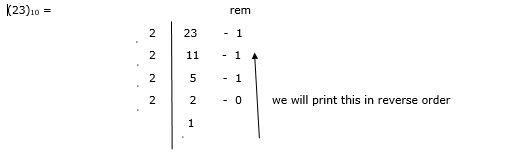# Java Program for Decimal to Binary Conversion

The number system is of four types: Binary, Octal, Decimal and Hexadecimal with base value 2, 8, 10 and 16 respectively. The base value depends on the number of digits contained by number system. For example, hexadecimal number system contains 16 digits i.e. 0 to 9 and A to F. Hence, its base is 16.

In this article, we will discuss the binary and decimal number systems. Also, we will make java programs to convert decimal to binary numbers.

## Binary and Decimal number system

### Binary number system

Binary number system works in the form of 0s and 1s. Since it has only two digits 0 and 1 therefore, its base is 2. In terms of electrical signal these two bits represent true and false or on and off states. (101)2, (011)2, (111)2 are a few examples of binary number.

Generally, it is used in digital devices like computers, smartphones, smartwatches. In fact, it is the base of all the operations performed on digital devices.

### Decimal number system

It is the most used number system. It has 10 digits from 0 to 9. Hence, its base value is 10. If base value of a number is not mentioned then, it is considered to be 10. A single digit has a weight of power 10 and every digit is 10 times more weighty than previous one. For example, 1010, 43110, 98010 etc.

The following table represents the binary and decimal formats of first 15 numbers −

Binary

Decimal

0001

1

0010

2

0011

3

0100

4

0101

5

0110

6

0111

7

1000

8

1001

9

1010

10

1011

11

1100

12

1101

13

1110

14

1111

15

In the above table, Binary number is represented in 4-bit format.

## Decimal to Binary Conversion

Let’s understand how we can convert decimal number to binary.

We convert the decimal format to binary format by dividing the decimal number until we get the quotient as 1 by the base of binary i.e. 2 . In other words, we will divide the given decimal number till the number is greater than 0.

### Example 1

Convert decimal number (23)10 to its binary form.Now, we will see the java program in which we will apply the above logic to convert hexadecimal to decimal.

### Approach 1: Using toBinaryString() method

It is a static method of ‘Integer’ class that returns a string value according to the base 2. It is available in java.lang package.

### Syntax

Integer.toBinaryString(int var_to_convert);


### Parameters

• Var_to_convert: the value that is going to be converted.

In the following approach, we will create a constructor ‘Cnvrt()’ along with a parameter ‘dec’ of integer type. In this constructor, we will perform the conversion and store the value in a string ‘b1’. In the main method, we will create an object of class ‘Cnvrt’ so that we can call the constructor with different values.

### Example

public class Cnvrt {
String Cnvrt(int dec) {
// Constructor
String b1 = Integer.toBinaryString(dec);
return b1;
// returing the string stored in b1
}
public static void main(String args[]) {
Cnvrt obj = new Cnvrt();
// creating object ‘obj’
// calling the constructor using object with arguments
System.out.println("Binary value of given decimal number: " + obj.Cnvrt(50));
System.out.println("Binary value of given decimal number: " + obj.Cnvrt(25));
System.out.println("Binary value of given decimal number: " + obj.Cnvrt(10));
}
}


### Output

Binary value of given decimal number: 110010
Binary value of given decimal number: 11001
Binary value of given decimal number: 1010


### Approach 2: Using our custom logic

In this approach, we will create four integer variables −

• ‘dec’ with a decimal value

• ‘b1’ to store converted binary number

• ‘rem’ to store the remainder

• ‘rev’ will be used while printing the remainders in reverse order

We will take a while loop and run it till the decimal value becomes 1. In this loop, we will divide and store the remainders. After that we will reverse the remainders.

### Example

public class Cnvrt {
public static void main(String[] args) {
int dec = 23;
int b1 = 0;
int rem;
int rev = 1;
while (dec > 0) {
rem = dec % 2;
// storing remainder
dec = dec / 2;
// dividing the given decimal value
b1 = b1 + rem * rev;
// reversing the remainders and storing it
rev = rev * 10;
}
System.out.println("Binary value of given decimal number: " + b1);
}
}


### Output

Binary value of given decimal number: 10111


## Conclusion

In this article, we have understood the types of number systems specifically binary and decimal number systems. Also, we discussed two approaches for making java program to convert decimal numbers to binary numbers.

Updated on: 02-May-2023

2K+ Views